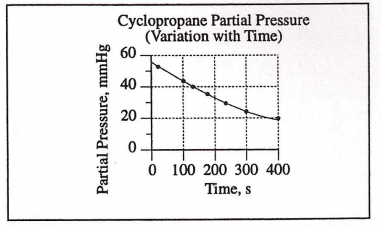# Problem: The rate of conversion from cyclopropane to propene in the gas phase at constant temperature is a first order reaction. From the information in the graph, which statement about the half-life of the conversion reaction is true?(A) It is approximately equal to 150 s.(B) It is approximately equal to 270 s.(C) It is approximately equal to 420 s.(D) It cannot be determined.

87% (24 ratings)
###### Problem Details

The rate of conversion from cyclopropane to propene in the gas phase at constant temperature is a first order reaction. From the information in the graph, which statement about the half-life of the conversion reaction is true?

(A) It is approximately equal to 150 s.

(B) It is approximately equal to 270 s.

(C) It is approximately equal to 420 s.

(D) It cannot be determined.What scientific concept do you need to know in order to solve this problem?

Our tutors have indicated that to solve this problem you will need to apply the Integrated Rate Law concept. You can view video lessons to learn Integrated Rate Law. Or if you need more Integrated Rate Law practice, you can also practice Integrated Rate Law practice problems.

What is the difficulty of this problem?

Our tutors rated the difficulty ofThe rate of conversion from cyclopropane to propene in the g...as medium difficulty.

How long does this problem take to solve?

Our expert Chemistry tutor, Dasha took 2 minutes and 15 seconds to solve this problem. You can follow their steps in the video explanation above.

What professor is this problem relevant for?

Based on our data, we think this problem is relevant for Professor Hampton's class at UCF.